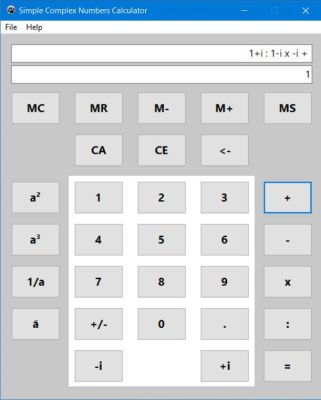# Computing: Free Pascal Programming

 Programming Home Home Contact

## Simple complex numbers calculator (SimpleCplxCalculator).

Description: Very simple calculator application, that may be used to perform basic arithmetic operations (add, subtract, multiply, divide) with complex numbers.

To enter a complex number a = x+yi, proceed as follows:

1. Enter its real part x, just as you would enter any real number.
2. Start entering the imaginary part by pushing the "+i" or "-i" button (depending on the imaginary part's sign).
3. Enter the imaginary part y (as you would do for a positive real number).
The sign of the complexes real part may be changed, using the "+/-" button. To change the sign of the imaginary part, you may use the conjugate function ("ā" button).

"Memory" and "Clear" buttons work exactly the same way as they do on a standard calculator.

Possible extensions: Make the application a "real" calculator by adding functions, complex format conversion...